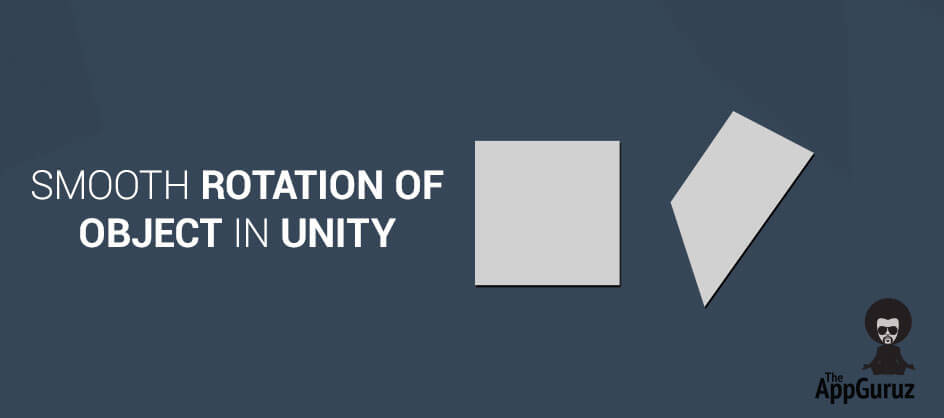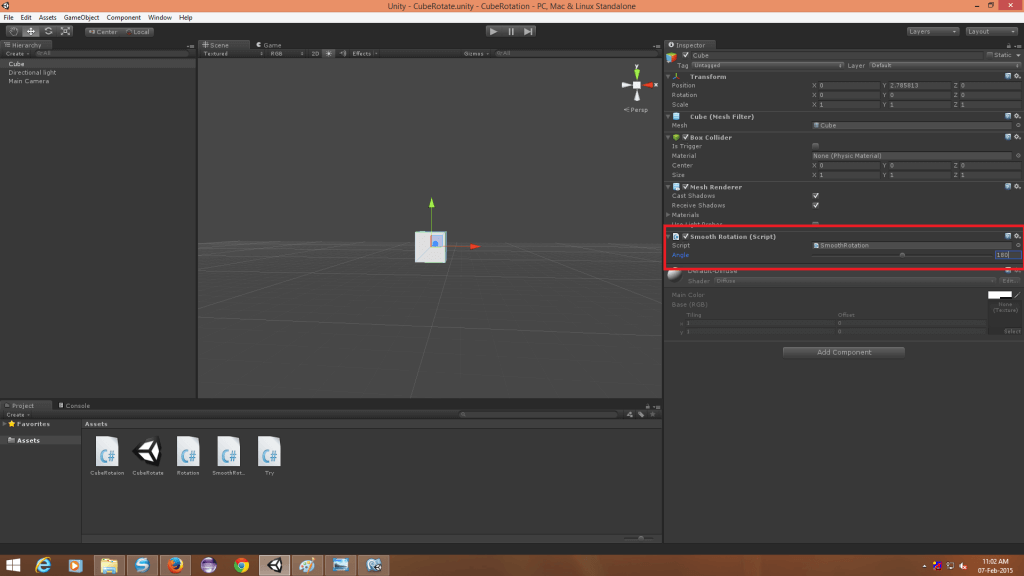## Objectives

The main objective of this blog post is to explain how to rotate any gameObject smoothly without any animation. Smooth Rotation of Object Tutorial

## Step 1 Project Setup

Add a game object in the hierarchy that you want to rotate. Add SmoothRotation script to that object.Set an angle at which you want to rotate the object.

## Step 2 Code Sample

### 2.1 SmoothRotation Script

``````using UnityEngine;
using System.Collections;

publicclassSmoothRotation : MonoBehaviour {

[Range(0,360)]publicfloat angle;//Specify Angle For Rotation
float tempAngle;//Temporary Angle Measurement Variable
bool horizontalFlag;//Check For Horizontal Roation
bool verticalFlag;//Check For Vertical Roation
bool isRotating;//Check Whether Currently Object is Rotating Or Not.
int Direction;//Direction Of Rotation

//Called For Initialization
void Start () {
horizontalFlag=verticalFlag=isRotating=false;
}

//Method For Horizontal Input
void CheckForHorizontalInput()
{
if(Input.GetAxis("Horizontal")!=0 && !isRotating)
{
isRotating=true;
Direction=(Input.GetAxis("Horizontal")<0?-1:1);
horizontalFlag=true;
tempAngle=0;
}
}

//Method For Vertical Input
void CheckForVerticalInput()
{
if(Input.GetAxis("Vertical")!=0 && !isRotating)
{
isRotating=true;
Direction=(Input.GetAxis("Vertical")<0?-1:1);
verticalFlag=true;
tempAngle=0;
}
}

//Method For horizontal Rotation
void HorizontalRotation()
{
transform.Rotate(Vector3.up*angle*Time.fixedDeltaTime*Direction,Space.World);
tempAngle+=angle*Time.fixedDeltaTime;
if(tempAngle>=angle)
{
tempAngle=0;
isRotating=false;
horizontalFlag=false;
}
}

//Method For Vertical Rotation
void VerticalRotation()
{
transform.Rotate(Vector3.right*angle*Time.fixedDeltaTime*Direction,Space.World);
tempAngle+=angle*Time.fixedDeltaTime;
if(tempAngle>=angle)
{
tempAngle=0;
isRotating=false;
verticalFlag=false;
}
}

void Update () {

CheckForHorizontalInput();
CheckForVerticalInput();
if(horizontalFlag)
HorizontalRotation();
if(verticalFlag)
VerticalRotation();

}
}``````

### 2.2 void CheckForHorizontalInput()

- If the object is not rotating currently, it will get a horizontal input from the user (Left/Right).

- Set horizontal flag to true.

- According to the input, set direction for rotation.

 Direction 1 for right direction Direction -1 for left direction

### 2.3 void CheckForVerticalInput()

- If the object is not rotating currently, it will get a vertical input from the user (Up/Down).

- Set vertical flag to true.

- According to the input, set direction for rotation.

 Direction 1 for up direction Direction -1 for down direction

### 2.4 void HorizontalRotation()

- It will rotate the object according to the direction until tempAngle is less then angle.

- Once the object is set to target rotation, it will stop rotating and will set isRotating to false.

Set tempAngle to 0.

Set horizontalFlag to false.

### 2.5 void VerticalRotation()

- It will rotate the object according to the direction until tempAngle is less then angle.

- Once the object is set to target rotation, it will stop rotating and will set isRotating to false.

Set tempAngle to 0.

Set verticalFlag to false.

### 2.6 void Update()

- Continuous check for horizontal input.

- Continuous check for vertical input.

- If horizontalFlag becomes true, call HorizontalRotation().

- If verticalFlag becomes true, call VerticalRotation().

I hope you find this blog is very helpful while working with Smooth Rotation object in Unity. Let me know in comment if you have any question regarding Smooth Rotation Object.

Got an Idea of Game Development? What are you still waiting for? Contact us now and see the Idea live soon. Our company has been named as one of the best Game Development Company in India.I am professional Android App & Game developer. I love to develop unique and creative Games & Apps. I am very passionate about my work.